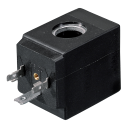Solenoid coil duty cycle explained

By Admin on Tue 23 January 2018The Duty Cycle of a coil is expressed as a percentage and represents the proportion of time that a solenoid is energised.

Duty cycle =        time off / (time on +time off)  x 100

If the energised period is continuous then the solenoid will need to be rated at 100%. However if a solenoid is energised for 15 seconds and switched "off" for 45 seconds before being energised again - then the total on/off cycle time is 60 seconds. This would be expressed as 25% duty cycle.

So as an example:       120 seconds on + 120 second off

120/( 120 +120) x 100

120/240 x 100 = 50%

60% would be:            120 seconds on

Time off = T

60 = 100 x 120/(120 + T)

A solenoid coil is normally rated at a duty cycle based on a standard ambient temperature of 35°C and a particular chosen voltage.

Most of our solenoid coils come with a standard 100% duty cycle which makes them ideal for most applications.